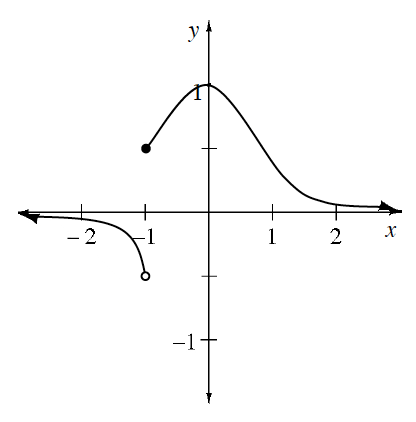### Home > APCALC > Chapter 2 > Lesson 2.2.1 > Problem2-52

2-52.

Write as many limit statements as you can about the function graphed at right as $x \rightarrow −1$ and $x \rightarrow ±\infty$.

Limits give information about the location of holes and asymptotes, because they are predicted values, not real values.

#### An Intuitive Definition of Limit

When you graph a function $y=f(x)$, most of the time you can guess what the value of, say, $f(3)$ is by knowing the values of $f(x)$ when $x$ is very close to $3$. One way to think about this is to assume you have the graph $y=f(x)$ for $2, except at $x=3$. Can you make a reasonable accurate guess as to the value of  $f(3)$? If so, and this value is $L$, we say that the limit of $f(x)$ exists at $x=3$ and use the notation  $\lim\limits _ { x \rightarrow 3 } f ( x ) = L$.

For example, if $g(3.01) = 4.02, g(3.001) = 4.005$, and $g(2.999) = 3.997$, it is reasonable to guess that $g(3) = 4$ and therefore $\lim\limits_ { x \rightarrow 3 } g ( x ) = 4$.

You can also take one-sided limits using numbers less than $a$ (the notation is $\lim\limits_ { x \rightarrow a ^ { - } } f ( x )$) or greater than $a$ (the notation is $\lim\limits_{ x \rightarrow a ^ { + } } f ( x )$).

An important point is that $\lim\limits_ { x \rightarrow a } f ( x )$does not need to equal $f (a)$.

$\lim\limits_{x\rightarrow 1^{-}}f(x)=$
$\lim\limits_{x\rightarrow 1^{+}}f(x)=$
$\lim\limits_{x\rightarrow -\infty }f(x)=$
$\lim\limits_{x\rightarrow \infty }f(x)=$